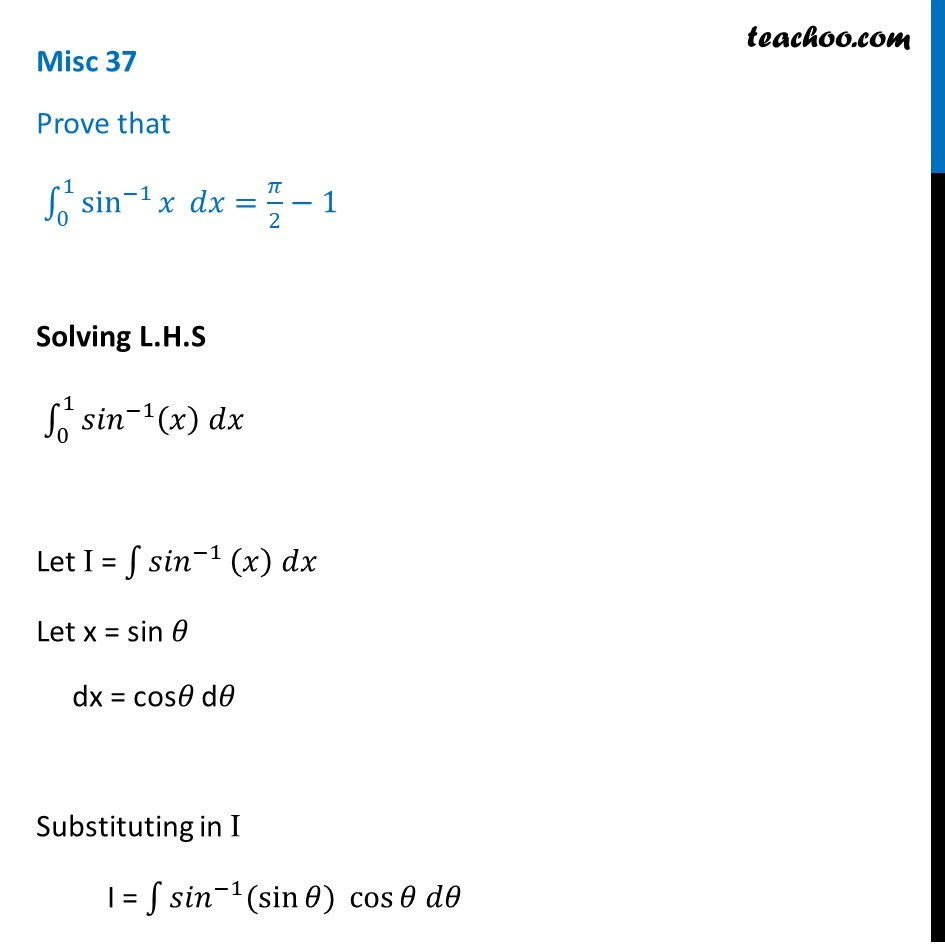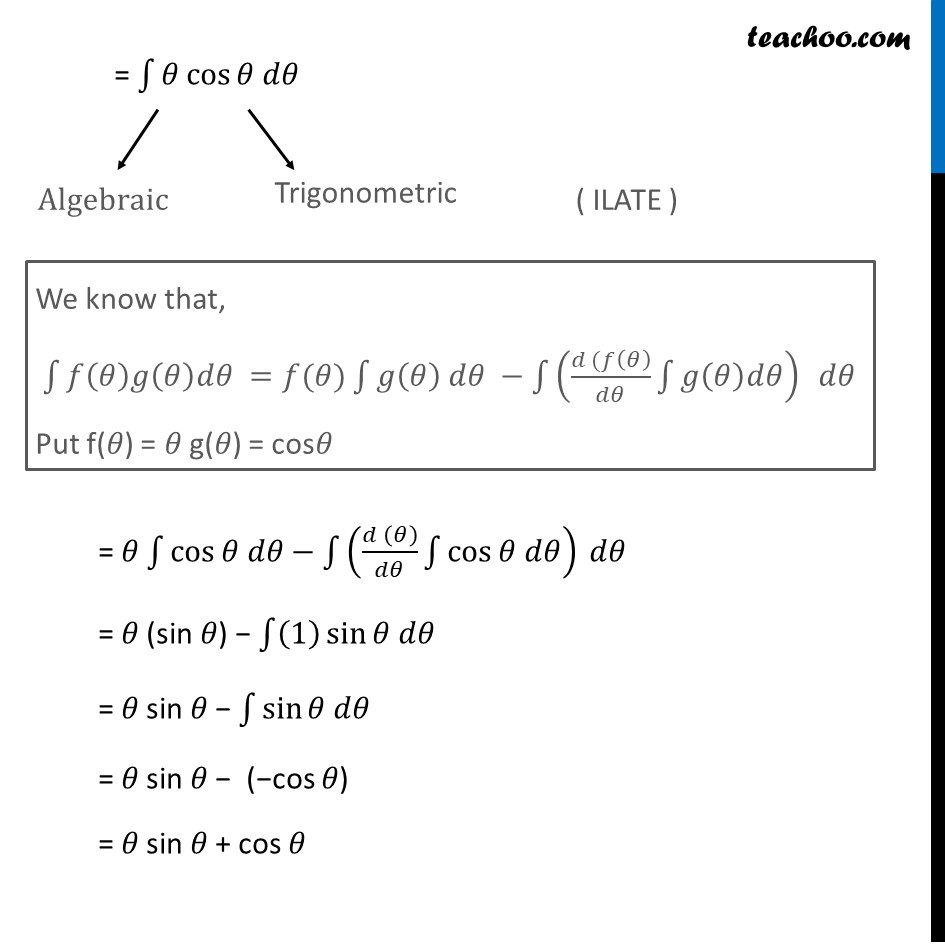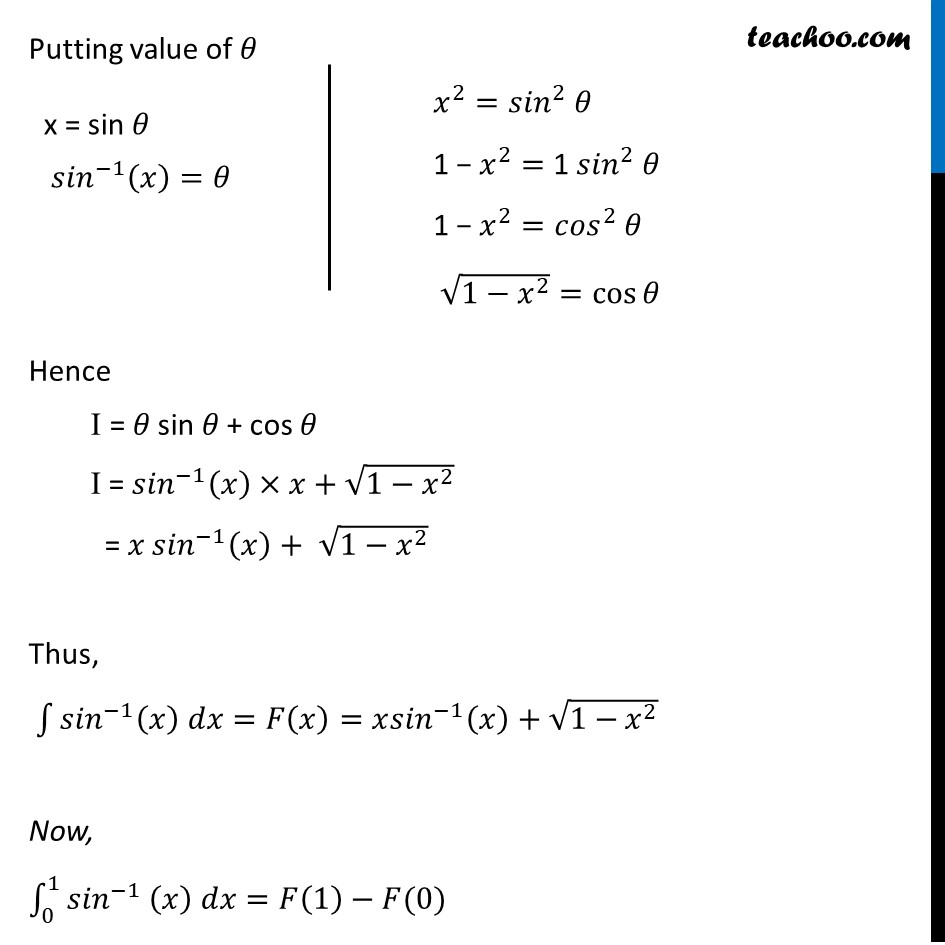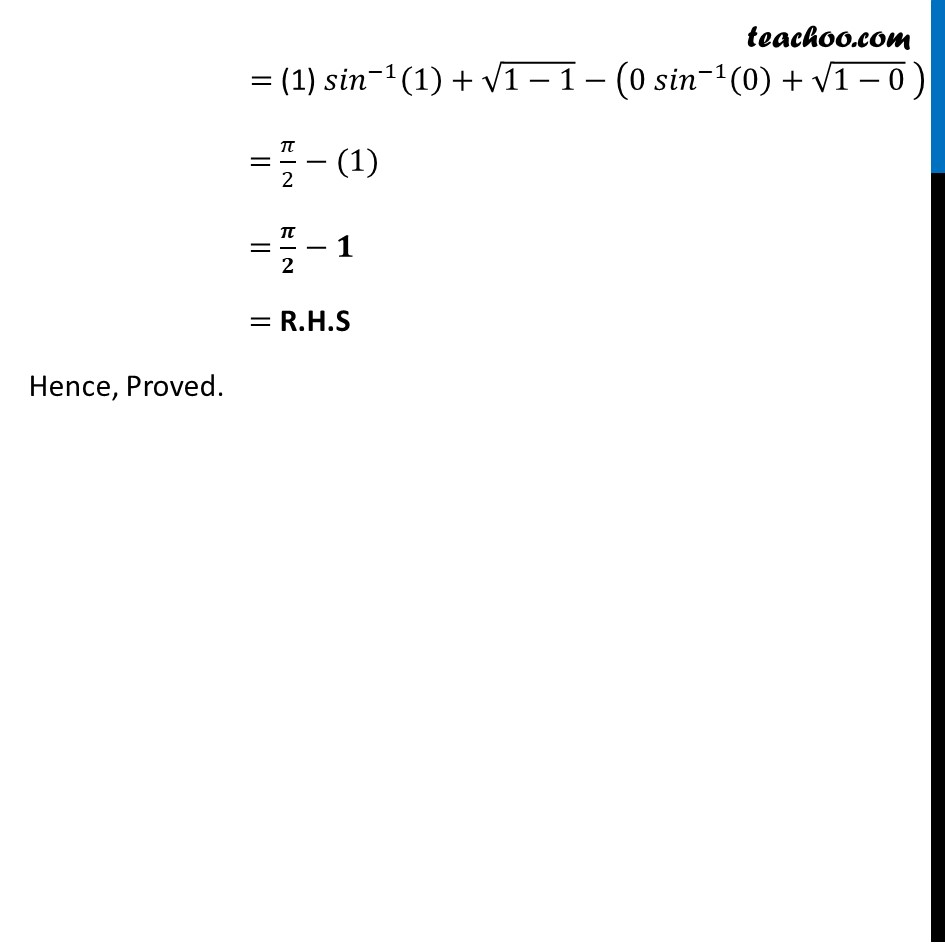Miscellaneous

Chapter 7 Class 12 Integrals
Serial order wiseLearn in your speed, with individual attention - Teachoo Maths 1-on-1 Class

### Transcript

Misc 37 Prove that ∫_0^1▒sin^(−1)⁡𝑥 𝑑𝑥=𝜋/2−1 Solving L.H.S ∫_0^1▒〖〖𝑠𝑖𝑛〗^(−1) (𝑥) 𝑑𝑥〗 Let I = ∫1▒〖𝑠𝑖𝑛〗^(−1) (𝑥) 𝑑𝑥 Let x = sin 𝜃 dx = cos𝜃 d𝜃 Substituting in I I = ∫1▒〖〖𝑠𝑖𝑛〗^(−1) (sin⁡〖𝜃) cos⁡〖𝜃 𝑑𝜃〗 〗 〗 = ∫1▒〖𝜃 cos⁡〖𝜃 𝑑𝜃〗 〗 = 𝜃 ∫1▒cos⁡〖𝜃 𝑑𝜃−∫1▒((𝑑 (𝜃))/𝑑𝜃 ∫1▒cos⁡〖𝜃 𝑑𝜃〗 ) 𝑑𝜃〗 = 𝜃 (sin 𝜃) − ∫1▒〖(1) sin⁡〖𝜃 𝑑𝜃〗 〗 = 𝜃 sin 𝜃 − ∫1▒sin⁡〖𝜃 𝑑𝜃〗 = 𝜃 sin 𝜃 − (−cos 𝜃) = 𝜃 sin 𝜃 + cos 𝜃 Putting value of 𝜃 Hence I = 𝜃 sin 𝜃 + cos 𝜃 I = 〖𝑠𝑖𝑛〗^(−1) (𝑥)×𝑥+√(1−𝑥^2 ) = 𝑥 〖𝑠𝑖𝑛〗^(−1) (𝑥)+ √(1−𝑥^2 ) Thus, ∫1▒〖〖𝑠𝑖𝑛〗^(−1) (𝑥) 𝑑𝑥=𝐹(𝑥)=𝑥〖𝑠𝑖𝑛〗^(−1) (𝑥)+√(1−𝑥^2 )〗 Now, ∫1_0^1▒〖〖𝑠𝑖𝑛〗^(−1) (𝑥) 𝑑𝑥=𝐹(1)−𝐹(0)〗 = (1) 〖𝑠𝑖𝑛〗^(−1) (1)+√(1−1)−(0〖 𝑠𝑖𝑛〗^(−1) (0)+√(1−0) ) =𝜋/2−(1) =𝝅/𝟐−𝟏 = R.H.S Hence, Proved.